# How to use INDIRECT function in Excel - formula examples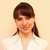by , updated on

This Excel INDIRECT tutorial explains the function's syntax, basic uses and provides a number of formula examples that demonstrate how to use INDIRECT in Excel.

A great lot of functions exist in Microsoft Excel, some being easy-to-understand, other requiring a long learning curve, and the former being used more often than the latter. And yet, Excel INDIRECT is one of the kind. This Excel function does not perform any calculations, nor does it evaluate any conditions or logical tests.

Well then, what is the INDIRECT function in Excel and what do I use it for? This is a very good question and hopefully you will get a comprehensive answer in a few minutes when you've finished reading this tutorial.

## Excel INDIRECT function - syntax and basic uses

As its name suggests, Excel INDIRECT is used to indirectly reference cells, ranges, other sheets or workbooks. In other words, the INDIRECT function lets you create a dynamic cell or range reference instead of hard-coding them. As a result, you can change a reference within a formula without changing the formula itself. Moreover, these indirect references won't change when some new rows or columns are inserted in the worksheet or when you delete any existing ones.

All this may be easier to understand from an example. However, to be able to write a formula, even the simplest one, you need to know the function's arguments, right? So, let's have a quick look at Excel INDIRECT syntax first.

#### INDIRECT function syntax

The INDIRECT function in Excel returns a cell reference from a text string. It has two arguments, the first is required and the second is optional:

INDIRECT(ref_text, [a1])

ref_text - is a cell reference, or a reference to a cell in the form of a text string, or a named range.

a1 - is a logical value that specifies what type of reference is contained in the ref_text argument:

• If TRUE or omitted, ref_text is interpreted as an A1-style cell reference.
• If FALSE, ref_text is treated as a R1C1 reference.

While the R1C1 reference type might be useful in certain situations, you'll probably want to use the familiar A1 references most of the time. Anyway, nearly all INDIRECT formulas in this tutorial will use A1 references, so we will be omitting the second argument.

#### Basic use of the INDIRECT function

To get into the function's insight, let's write a simple formula that demonstrates how you use INDIRECT in Excel.

Suppose, you have number 3 in cell A1, and text A1 in cell C1. Now, put the formula `=INDIRECT(C1)` in any other cell and see what happens:

• The INDIRECT function refers to the value in cell C1, which is A1.
• The function is routed to cell A1 where it picks the value to return, which is number 3.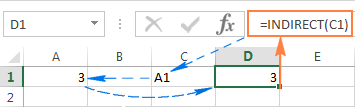So, what the INDIRECT function actually does in this example is converting a text string into a cell reference.

If you think this still has very little practical sense, please bear with me and I will show you some more formulas that reveal the real power of the Excel INDIRECT function.

## How to use INDIRECT in Excel - formula examples

As demonstrated in the above example, you can use the Excel INDIRECT function to put the address of one cell into another as a usual text string, and get the value of the 1st cell by referencing the 2nd. However, that trivial example is no more than a hint at the INDIRECT capabilities.

When working with real data, the INDIRECT function can turn any text string into a reference including very complex strings that you build using the values of other cells and results returned by other Excel formulas. But let's not put the cart before the horse, and run through several Excel Indirect formulas, one at a time.

### Creating indirect references from cell values

As you remember, the Excel INDIRECT function allows for A1 and R1C1 reference styles. Usually, you cannot use both styles in a single sheet at a time, you can only switch between the two reference types via File > Options > Formulas > R1C1 check box. This is the reason why Excel users rarely consider using R1C1 as an alternative referencing approach.

In an INDIRECT formula, you can use either reference type on the same sheet if you want to. Before we move any further, you may want to know the difference between A1 and R1C1 reference styles.

A1 style is the usual reference type in Excel that refers to a column followed by a row number. For example, B2 refers to the cell at the intersection of column B and row 2.

R1C1 style is the opposite reference type - rows followed by columns, which does take some time getting used to : ) For example, R4C1 refers to cell A4 which is in row 4, column 1 in a sheet. If no number comes after the letter, then you are referring to the same row or column.

And now, let's see how the INDIRECT function handles A1 and R1C1 references: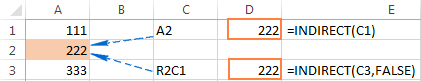As you see in the screenshot above, three different Indirect formulas return the same result. Have you already figured out why? I bet you have : )

• Formula in cell D1: `=INDIRECT(C1)`

This is the easiest one. The formula refers to cell C1, fetches its value - text string A2, converts it into a cell reference, heads over to cell A2 and returns its value, which is 222.

• Formula in cell D3: `=INDIRECT(C3,FALSE)`

FALSE in the 2nd argument indicates that the referred value (C3) should be treated like a R1C1 cell reference, i.e. a row number followed by a column number. Therefore, our INDIRECT formula interprets the value in cell C3 (R2C1) as a reference to the cell at the conjunction of row 2 and column 1, which is cell A2.

### Creating indirect references from cell values and text

Similarly to how we created references from cell values, you can combine a text string and a cell reference within your INDIRECT formula, tied together with the concatenation operator (&).

In the following example, the formula: =INDIRECT("B"&C2) returns a value from cell B2 based on the following logical chain:

The INDIRECT function concatenates the elements in the ref_text argument - text B and the value in cell C2 -> the value in cell C2 is number 2, which makes a reference to cell B2 -> the formula goes to cell B2 and returns its value, which is number 10.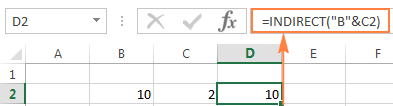### Using the INDIRECT function with named ranges

Apart from making references from cell and text values, you can get the Excel INDIRECT function to refer to named ranges.

Suppose, you have the following named ranges in your sheet:

• Apples - B2:B6
• Bananas - C2:C6
• Lemons - D2:D6

To create an Excel dynamic reference to any of the above named ranges, just enter its name in some cell, say G1, and refer to that cell from an Indirect formula `=INDIRECT(G1)`.

And now, you can take a step further and imbed this INDIRECT formula into other Excel functions to calculate the sum and average of the values in a given named range, or find the maximum / minimum value within the rage:

• `=SUM(INDIRECT(G1))`
• `=AVERAGE(INDIRECT(G1))`
• `=MAX(INDIRECT(G1))`
• `=MIN(INDIRECT(G1))`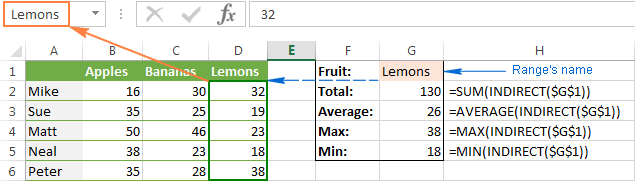Now that you've got the general idea of how to use the INDIRECT function in Excel, we can experiment with more powerful formulas.

### INDIRECT formula to dynamically refer to another worksheet

The usefulness of the Excel INDIRECT function is not limited to building "dynamic" cell references. You can also utilize it to refer to cells in other worksheets "on the fly", and here's how.

Suppose, you have some important data in Sheet 1, and you want to pull that data in Sheet 2. The following screenshot demonstrates how an Excel Indirect formula can handle this task: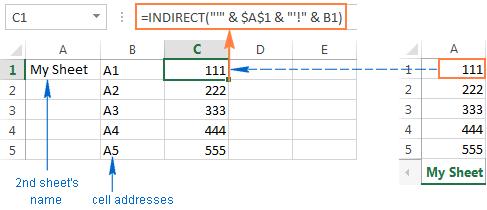Let's break apart the formula you see in the screenshot and understand.

As you know, the usual way to reference another sheet in Excel is writing the sheet's name followed by the exclamation mark and a cell / range reference, like SheetName!Range. Since a sheet name often contains a space(s), you'd better enclose it (the name, not a space : ) in single quotes to prevent an error, for example 'My Sheet!'\$A\$1.

And now, all you have to do is to enter the sheet name in one cell, the cell address in another, concatenate them in a text string, and feed that string to the INDIRECT function. Remember that in a text string, you have to enclose each element other than a cell address or number in double quotes and link all elements together using the concatenation operator (&).

Given the above, we get the following pattern:

INDIRECT("'" & Sheet's name & "'!" & Cell to pull data from)

Going back to our example, you put the sheet's name in cell A1, and type the cell addresses in column B, as demonstrated in the screenshot above. As the result, you get the following formula:

`INDIRECT("'" & \$A\$1 & "'!" & B1)`

Also, please pay attention that if you are copying the formula into multiple cells, you have to lock the reference to the sheet's name using the absolute cell references like \$A\$1.

Notes

• If either of the cells that contain the 2nd sheet's name and cell address (A1 and B1 in the above formula) is empty, your Indirect formula will return an error. To prevent this, you can wrap the INDIRECT function in the IF function:

`IF(OR(\$A\$1="",B1=""), "", INDIRECT("'" & \$A\$1 & "'!" & B1))`

• For the INDIRECT formula that refers to another sheet to work correctly, the referred sheet should be open, otherwise the formula will return a #REF error. To avoid the error, you can use the IFERROR function, which will display an empty string, whatever error occurs:

`IFERROR(INDIRECT("'" & \$A\$1 & "'!" &B1), "")`

### Creating an Excel dynamic reference to another workbook

The Indirect formula that refers to a different Excel workbook is based on the same approach as a reference to another spreadsheet. You just have to specify the workbook's name is addition to the sheet name and cell address.

To make things easier, let's start with making a reference to another book in the usual way (apostrophes are added in case your book and/or sheet names contain spaces):
'[Book_name.xlsx]Sheet_name'!Range

Assuming that the book name is in cell A2, the sheet name is in B2, and the cell address is in C2, we get the following formula:

`=INDIRECT("'[" & \$A\$2 & "]" & \$B\$2 & "'!" & C2)`

Since you don't want the cells containing the book's and sheet's names to change when copying the formula to other cells, you lock them by using absolute cell references, \$A\$2 and \$B\$2, respectively.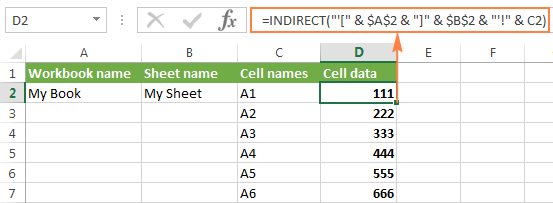And now, you can easily write your own dynamic reference to another Excel workbook by using the following pattern:

=INDIRECT("'[" & Book name & "]" & Sheet name & "'!" & Cell address)

Note. The workbook your formula refers to should always be open, otherwise the INDIRECT function will throw a #REF error. As usual, the IFERROR function can help you avoid it:

`=IFERROR(INDIRECT("'[" & A2 & "]" & \$A\$1 & "'!" & B1), "")`

### Using Excel INDIRECT function to lock a cell reference

Normally, Microsoft Excel changes cell references when you insert new or delete existing rows or columns in a sheet. To prevent this from happening, you can use the INDIRECT function to work with cell references that should remain intact in any case.

To illustrate the difference, please do the following:

1. Enter any value in any cell, say, number 20 in cell A1.
2. Refer to A1 from two other cells in different ways: `=A1` and `=INDIRECT("A1")`
3. Insert a new row above row 1.

See what happens? The cell with the equal to logical operator still returns 20, because its formula has been automatically changed to =A2. The cell with the INDIRECT formula now returns 0, because the formula was not changed when a new row was inserted and it still refers to cell A1, which is currently empty: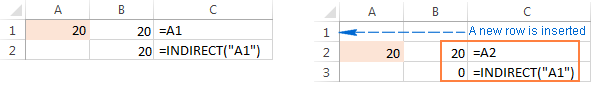After this demonstration, you may be under the impression that the INDIRECT function is more a nuisance than help. Okay, let's try it another way.

Suppose, you want to sum the values in cells A2:A5, and you can easily do this using the SUM function:

`=SUM(A2:A5)`

However, you want the formula to remain unchanged, no matter how many rows are deleted or inserted. The most obvious solution - the use of absolute references - won't help. To make sure, enter the formula `=SUM(\$A\$2:\$A\$5)` in some cell, insert a new row, say at row 3, and… find the formula converted to `=SUM(\$A\$2:\$A\$6)`.

Of course, such courtesy of Microsoft Excel will work fine in most cases. Nevertheless, there may be scenarios when you don't want the formula to get changed automatically. The solution is to use the INDIRECT function, like this:

`=SUM(INDIRECT("A2:A5"))`

Since Excel perceives "A1:A5" as a mere text string rather than a range reference, it won't make any changes when you insert or delete a row(s).

### Using INDIRECT with other Excel functions

Apart from SUM, INDIRECT is frequently used with other Excel functions such as ROW, COLUMN, ADDRESS, VLOOKUP, SUMIF, to name a few.

#### Example 1. INDIRECT and ROW functions

Quite often, the ROW function is used in Excel to return an array of values. For example, you can use the following array formula (remember it requires pressing Ctrl + Shift + Enter) to return the average of the 3 smallest numbers in the range A1:A10:

`=AVERAGE(SMALL(A1:A10,ROW(1:3)))`

However, if you insert a new row in your worksheet, anywhere between rows 1 and 3, the range in the ROW function will get changed to ROW(1:4) and the formula will return the average of the 4 smallest numbers instead of 3.

To prevent this from happening, nest INDIRECT in the ROW function and your array formula will always remain correct, no matter how many rows are inserted or deleted:

`=AVERAGE(SMALL(A1:A10,ROW(INDIRECT("1:3"))))`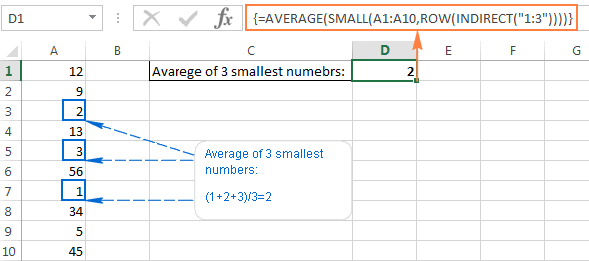Here's a couple more examples of using INDIRECT and ROW in conjunction with the LARGE function: How to sum N largest numbers in a range.

#### Example 2. INDIRECT and ADDRESS functions

You can use Excel INDIRECT together with the ADDRESS function to get a value in a certain cell on the fly.

As you may remember, the ADDRESS function is used in Excel to get a cell address by the row and column numbers. For example, the formula `=ADDRESS(1,3)` returns the string \$C\$1 since C1 is the cell at the intersection of the 1st row and 3rd column.

To create an indirect cell reference, you simply embed the ADDRESS function into an INDIRECT formula like this:

`=INDIRECT(ADDRESS(1,3))`

Of course, this trivial formula only demonstrates the technique. And here are a few examples that may prove really useful:

### Using INDIRECT with Data Validation in Excel

You can use the Excel INDIRECT function with Data Validation to create cascading drop down lists that display different choices depending on what value the user selected in the first dropdown.

A simple dependent drop-down list is really easy to make. All it takes is a few named ranges to store the dropdown's items and a simple `=INDIRECT(A2)` formula where A2 is the cell displaying your first drop-down list.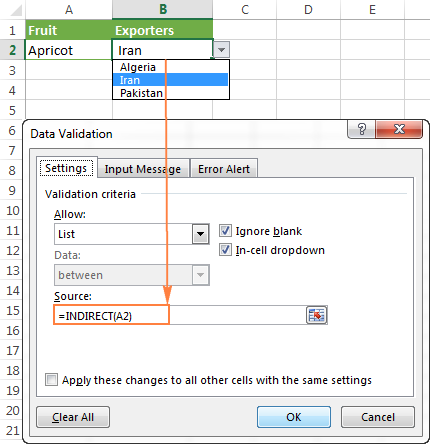To make more complex 3-level menus or drop-downs with multi-word entries, you will need a bit more complex INDIRECT formula with a nested SUBSTITUTE function.

For the detailed step-by-step guidance on how to use INDIRECT with Excel Data Validation, please check out this tutorial: How to make a dependent drop down list in Excel.

## Excel INDIRECT function - possible errors and issues

As demonstrated in the above examples, the INDIRECT function is quite helpful when dealing with cell and range references. However, not all Excel users eagerly embrace it mostly because extensive use of INDIRECT in Excel formulas results in lack of transparency. The INDIRECT function is difficult to review since the cell it refers to is not the ultimate location of the value used in the formula, which is pretty confusing indeed, especially when working with large complex formulas.

In addition to the above said, like any other Excel function, INDIRECT may throw an error if you misuse the function's arguments. Here's a list of most typical mistakes:

#### Excel INDIRECT #REF! error

Most often, the INDIRECT function returns a #REF! error in three cases:

1. ref_text is not a valid cell reference. If the ref_text parameter in your Indirect formula is not a valid cell reference, the formula will result in the #REF! error value. To avoid possible issues, please check the INDIRECT function's arguments.
2. Range limit is exceeded. If the ref_text argument of your Indirect formula refers to a range of cells beyond the row limit of 1,048,576 or the column limit of 16,384, you will also get the #REF error in Excel 2007, 2010 and Excel 2013. Earlier Excel versions ignore the exceeded limit and do return some value, though often not the one you'd expect.
3. The referred sheet or workbook is closed. If your Indirect formula refers to another Excel workbook or worksheet, that other workbook / spreadsheet must be open, otherwise INDIRECT returns the #REF! error.

#### Excel INDIRECT #NAME? error

This is the most obvious case, implying that there is some error in the function's name, which leads us to the next point : )

#### Using the INDIRECT function in non-English locales

You may be curious to know that the English name of the INDIRECT function has been translated into 14 languages, including:

 Danish - INDIREKTE Finnish - EPÄSUORA German - INDIREKT Hungarian - INDIREKT Italian - INDIRETTO Norwegian - INDIREKTE Polish - ADR.POŚR Spanish - INDIRECTO Swedish - INDIREKT Turkish - DOLAYLI